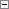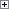This topic explains how items, such as documents, are numbered in Microsoft Dynamics NAV.

Number Sorting

Code fields in tables can contain both numeric values and text strings. By default, code fields are stored in Microsoft SQL Server using the nvarchar SQL data type. Nvarchar data types are sorted as if they are text strings. The following table illustrates the differences that occur in sorting as numeric values and sorting as text.

Numeric sorting Text sorting

1

1

2

10

3

100

4

2

10

3

100

4

You can change the SQL Data Type property to specify that a code field is stored as an Integer, BigInteger, or Variant instead of a Varchar. Storing a code field as an Integer, BigInteger, or Variant introduces additional constraints on the data in the field. For more information about these constraints, see SQL Data Type Property.

To avoid sorting problems with code fields, we recommend that you use a numeric series that has a fixed length. You can do this in the following ways:

• Define a numeric series that consists of a predefined number of digits that start with a digit other than zero. The following are examples of fixed length numeric series:
• 100-399 (300 numbers)
• 40000-69999 (30,000 numbers)
• 7000000-9999999 (3,000,000 numbers)
The values are sorted the same way whether they are sorted as characters or as numeric values.
This solution enables you to set the SQL Data Type property to Varchar, Integer, or BigInteger and the sorting is the same.
• Define a numeric series that consists of a predefined number of digits and that starts with a letter, such as A001-A999. When the series is complete, you can define a new series by starting with a different letter.
The values in this kind of series always begin with a letter and the sorting is always the same, regardless of the SQL data type.Important
As a rule, data types that are used in fields that are related to each other must be compatible. Therefore, when you use a SQL data type in a field, you will usually have to change the SQL data type settings of related fields in other tables. For example, in the general ledger application area, if you change the SQL data type of the No. field in the G/L Account table from Varchar to Integer (or if you change the data type from Code to Text), you must change the data type of the G/L Account No. fields in the G/L Entry and G/L Budget Entry tables to the corresponding data type. Failure to do this results in the display of incorrect totals, based on these tables, in the chart of accounts and elsewhere.

Filters

If you do not follow the numbering principles, problems will occur when you apply filters that involve numbers.

An example of this is if you have not used a numeric series that has a fixed length, when you apply a filter, for example, 10...20, the result will be 10,100...20.

When you follow the numbering principles, you must remember to use these principles for filters that you apply. Here are two examples:

• If you do not follow the numbering principles when you apply a filter, for example, 2...10, the result will contain no records. This is because 2, comes after 10.
• You have followed the numbering principles and are using three-digit numbers. If you forget to follow the same principles when you apply a filter, for example, 10...20, the result will be 100,101,102...199.